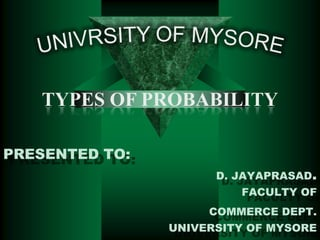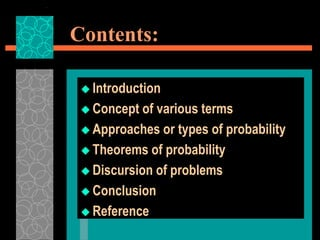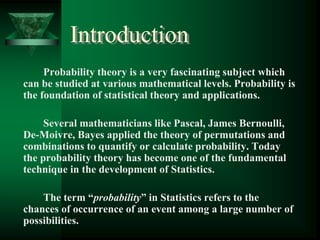Successfully reported this slideshow.

# PROBABILITY AND IT'S TYPES WITH RULES

71

Share×
1 of 20
1 of 20

# PROBABILITY AND IT'S TYPES WITH RULES

71

Share

## More Related Content

### Related Books

Free with a 14 day trial from Scribd

See all

### Related Audiobooks

Free with a 14 day trial from Scribd

See all

### PROBABILITY AND IT'S TYPES WITH RULES

1. 1. TYPES OF PROBABILITY PRESENTED TO: D. JAYAPRASAD. FACULTY OF COMMERCE DEPT. UNIVERSITY OF MYSORE
2. 2. Contents:  Introduction  Concept of various terms  Approaches or types of probability  Theorems of probability  Discursion of problems  Conclusion  Reference
3. 3. Introduction Probability theory is a very fascinating subject which can be studied at various mathematical levels. Probability is the foundation of statistical theory and applications. Several mathematicians like Pascal, James Bernoulli, De-Moivre, Bayes applied the theory of permutations and combinations to quantify or calculate probability. Today the probability theory has become one of the fundamental technique in the development of Statistics. The term “probability” in Statistics refers to the chances of occurrence of an event among a large number of possibilities.
4. 4. TERMINOLOGIES  Random Experiment: If an experiment or trial is repeated under the same conditions for any number of times and it is possible to count the total number of outcomes is called as “Random Experiment”.  Sample Space: The set of all possible outcomes of a random experiment is known as “Sample Space” and denoted by set S. [this is similar to Universal set in Set Theory] The outcomes of the random experiment are called sample points or outcomes.
5. 5. • Event: An „event‟ is an outcome of a trial meeting a specified set of conditions other words, event is a subset of the sample space S. Events are usually denoted by capital letters. There are different types of events. 1. Null or impossible event is an event which contains no outcomes. 2. Elementary event is an event which contains only one outcomes. 3. Composite event is an event which contains two or more outcomes. 4. Sure or certain event is an event which contains all the outcomes of a sample space.
6. 6. • Exhaustive Events: The total number of all possible elementary outcomes in a random experiment is known as „exhaustive events‟. In other words, a set is said to be exhaustive, when no other possibilities exists. • Favourable Events: The elementary outcomes which entail or favour the happening of an event is known as „favourable events‟ i.e., the outcomes which help in the occurrence of that event. • Mutually Exclusive Events: Events are said to be „mutually exclusive‟ if the occurrence of an event totally prevents occurrence of all other events in a trial. In other words, two events A and B cannot occur simultaneously.
7. 7. • Equally likely or Equi-probable Events: Outcomes are said to be ‘equally likely’ if there is no reason to expect one outcome to occur in preference to another. i.e., among all exhaustive outcomes, each of them has equal chance of occurrence. • Complementary Events: Let E denote occurrence of event. The complement of E denotes the non occurrence of event E. Complement of E is denoted by ‘Ē’. • Independent Events: Two or more events are said to be ‘independent’, in a series of a trials if the outcome of one event is does not affect the outcome of the other event or vise versa.
8. 8. In other words, several events are said to be „dependents‟ if the occurrence of an event is affected by the occurrence of any number of remaining events, in a series of trials. Measurement of Probability: There are three approaches to construct a measure of probability of occurrence of an event. They are:  Classical Approach,  Frequency Approach and  Axiomatic Approach.
9. 9. Classical or Mathematical Approach: In this approach we assume that an experiment or trial results in any one of many possible outcomes, each outcome being Equi-probable or equally-likely. Definition: If a trial results in „n‟ exhaustive, mutually exclusive, equally likely and independent outcomes, and if „m‟ of them are favourable for the happening of the event E, then the probability „P‟ of occurrence of the event „E‟ is given byNumber of outcomes favourable to event E m = P(E) = Exhaustive number of outcomes n
10. 10. Empirical or Statistical Approach: This approach is also called the „frequency‟ approach to probability. Here the probability is obtained by actually performing the experiment large number of times. As the number of trials n increases, we get more accurate result. Definition: Consider a random experiment which is repeated large number of times under essentially homogeneous and identical conditions. If „n‟ denotes the number of trials and „m‟ denotes the number of times an event A has occurred, then, probability of event A is the limiting value of the relative frequency m . n
11. 11. Axiomatic Approach: This approach was proposed by Russian Mathematician A.N.Kolmogorov in1933. „Axioms‟ are statements which are reasonably true and are accepted as such, without seeking any proof. Definition: Let S be the sample space associated with a random experiment. Let A be any event in S. then P(A) is the probability of occurrence of A if the following axioms are satisfied. 1. 2. 3. P(A)>0, where A is any event. P(S)=1. P(AUB) = P(A) + P(B), when event A and B are mutually exclusive.
12. 12. Three types of Probability 1. Theoretical probability: For theoretical reasons, we assume that all n possible outcomes of a particular experiment are equally likely, and we assign a probability of to each possible outcome. Example: The theoretical probability of rolling a 3 on a regular 6 sided die is 1/6
13. 13. 2. Relative frequency interpretation of probability: We conduct an experiment many, many times. Then we say The probability of event A = How many times A occurs How many trials Relative Frequency is based on observation or actual measurements. Example: A die is rolled 100 times. The number 3 is rolled 12 times. The relative frequency of rolling a 3 is 12/100. 3. Personal or subjective probability: These are values (between 0 and 1 or 0 and 100%) assigned by individuals based on how likely they think events are to occur. Example: The probability of my being asked on a date for this weekend is 10%.
14. 14. 1. The probability of an event is between 0 and 1. A probability of 1 is equivalent to 100% certainty. Probabilities can be expressed at fractions, decimals, or percents. 0 ≤ pr(A) ≤ 1  The sum of the probabilities of all possible outcomes is 1 or 100%. 2. If A, B, and C are the only possible outcomes, then pr(A) + pr(B) + pr(C) = 1 Example: A bag contains 5 red marbles, 3 blue marbles, and 2 green marbles. pr(red) + pr(blue) + pr(green) = 1 5 3 2 10 10 10 1 3. The sum of the probability of an event occurring and it not occurring is 1. pr(A) + pr(not A) = 1 or pr(not A) = 1 - pr(A) Example: A bag contains 5 red marbles, 3 blue marbles, and 2 green marbles. pr (red) + pr(not red) = 1 3 7 + pr(not red) = 1 10 pr(not red) = 10
15. 15. 4. If two events A and B are independent (this means that the occurrence of A has no impact at all on whether B occurs and vice versa), then the probability of A and B occurring is the product of their individual probabilities. pr (A and B) = pr(A) · pr(B) Example: roll a die and flip a coin. pr(heads and roll a 3) = pr(H) and pr(3) 1 1 1 2 6 12 5. If two events A and B are mutually exclusive (meaning A cannot occur at the same time as B occurs), then the probability of either A or B occurring is the sum of their individual probabilities. Pr(A or B) = pr(A) + pr(B) Example: A bag contains 5 red marbles, 3 blue marbles, and 2 green marbles. 5 2 7 10 10 10 pr(red or green) = pr(red) + pr(green) 6. If two events A and B are not mutually exclusive (meaning it is possible that A and B occur at the same time), then the probability of either A or B occurring is the sum of their individual probabilities minus the probability of both A and B occurring. Pr(A or B) = pr(A) + pr(B) – pr(A and B)
16. 16. Example: There are 20 people in the room: 12 girls (5 with blond hair and 7 with brown hair) and 8 boys (4 with blond hair and 4 with brown hair). There are a total of 9 blonds and 11 with brown hair. One person from the group is chosen randomly. pr(girl or blond) = pr(girl) + pr(blond) – pr(girl and blond) 12 9 5 16 20 20 20 20 7. The probability of at least one event occurring out of multiple events is equal to one minus the probability of none of the events occurring. pr(at least one) = 1 – pr(none) Example: roll a coin 4 times. What is the probability of getting at least one head on the 4 rolls. 1 1 1 1 pr(at least one H) = 1 – pr(no H) = 1 – pr (TTTT) = 1 2 2 2 2 1 15 =1 16 16 8. If event B is a subset of event A, then the probability of B is less than or equal to the probability of A. pr(B) ≤ pr(A) Example: There are 20 people in the room: 12 girls (5 with blond hair and 7 with brown hair) and 8 boys (4 with blond hair and 4 with brown hair). pr (girl with brown hair) ≤ pr(girl) 7 12 16 20 20
17. 17. Conclusion
18. 18. References  STATISTICS– Prof. HEMA RAVIKUMAR & Prof . MAMTHA RAMESH  Web References: www.google.com www.wekipedia.com
19. 19. PRESENTED BY • BHARGAVI.B. • M.F.M. Previous Year • University Of Mysore.
20. 20. THANK YOU